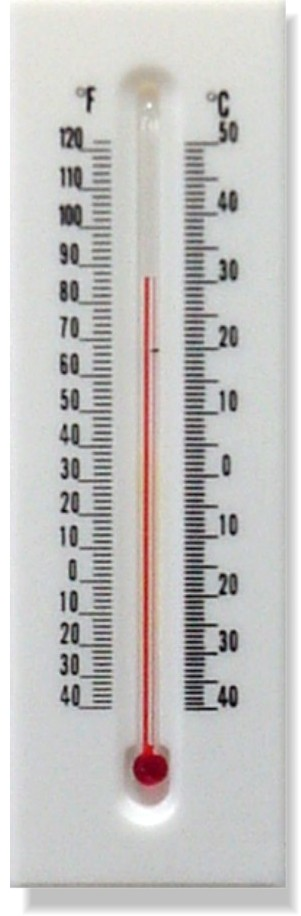Home > Grade 6 > Converting Between Fahrenheit And Celsius

# Converting Between Fahrenheit And Celsius

Directions: When is the value of the temperature in Fahrenheit the same as the value of the temperature in Celsius?

### Hint

How can we demonstrate this using numbers (a table of values), pictures (looking at a thermometer that uses both units), algebra, and words?

The answer is -40º.  In addition to using a table of values or algebra, it can be demonstrated by looking at images of real thermometers like this one:Source: Robert Kaplinsky

## Order of Operations 6

Directions: Using the digits 1 to 5, at most one time each, place a digit …

1.•